# The Tiger's Stripes

A technical blog on math, computer science, and game theory.

Author: Bo Waggoner RSS feed# Risk Aversion and Decisionmaking

Posted: 2017-09-28.

In this post, I want to review the basics of risk aversion for you, then argue that risk-aversion naturally arises in any situation where agents face a decision under uncertainty.

A risk-neutral agent in a decisionmaking context becomes risk averse.

First, we'll take a quick tutorial on risk aversion to see how it is mathematically modeled as concave "utility functions" mapping money to utility. Then, we'll show that these are equivalent to convex "certainty equivalent functions" mapping a probability distribution over outcomes to a monetary value. Finally, we'll see how facing a decision can cause risk-averse-like preferences. One intuition for this will be that both decisionmakers and risk-averse agents have positive value for information.

## A Happy Meal is a Gamble

It seems reasonable to suppose that people have preferences over things that happen. I prefer winning the race to coming in second; I prefer getting a Star Wars toy with my Happy Meal over a Star Trek toy.

But what about preferences over different random events? For instance, what about a 50-50 chance of each toy versus a 70-30?

We can formalize such scenarios as lotteries or "gambles": probability distributions over outcomes. Von Neumann and Morgenstern showed that, as long as an agent's preferences over lotteries satisfies some natural axioms, they can be expressed in a very simple way: assign a number to each outcome (e.g. Star Wars Toy = 10, Star Trek toy = 4), and for any lottery on the outcomes (such as 50-50), rank that lottery according to its expectation (such as $0.5 (10) + 0.5 (4) = 7$).

Here, the numbers assigned to the outcomes represent the "utility" of each outcome, and the expectation is the "expected utility" of the gamble.

If an agent satisfies the VNM axioms, then she ranks all possible lotteries in order of preference by their expected utility. So for instance, suppose I get utility 7 from watching an episode of Spongebob Squarepants. Then I am exactly indifferent between watching the episode for sure and taking the 50-50 Happy Meal lottery.

## What is Risk?

Risk refers to uncertainty over outcomes of varying quality or value. Being randomly assigned either strawberries or blueberries is not risky to me, because I like both equally. Getting my monthly internet bill isn't risky either; it's a negative, but it's certain to happen.

A Happy Meal, on the other hand - that's risky. I could end up with anything from the awesome Chewbacca to lame old Spock.

Now if you live in the real world, you might notice that a lot of people out there dislike risk. In fact, they dislike it so much they do things like buy insurance (spending much more on average than they get back) or eat at the same boring old restaurant every week rather than try something new.

But formalizing this mathematically is a bit tricky if we want to keep the VNM representation, because agents just evaluate lotteries based on expected utility, whether they're a sure bet (like the Spongebob episode) or "risky" (like the Happy Meal).

## Risk and Utility Functions

Economists typically formalize and quantify risk by translating utility into money. We have already formalized a gamble, such as receiving a Happy Meal toy, as a distribution over outcomes of varying utility. Now suppose that I assign a monetary value to each possible outcome as well.

This can be interpreted as saying that I have a utility function $u: \$ \to \mathbb{R}$mapping any dollar amount to the equivalent amount of utility. (I use the dollar sign to emphasize the units of the input, although it's just a real number.) A natural axiom we require$u$to satisfy is monotonicity: more money leads to more utility. Going further, for any lottery$p$, I would therefore assign an amount of money$CE^u(p)$to it. This is called the certainty equivalent and is the amount of money such that I would be indifferent between taking the money and taking the lottery. Example. Suppose my utility function is$u(m) = \sqrt{m}$. Then I get the following table. "Certainty equivalent" means the amount of money such that I would be indifferent between the money and the lottery. Lottery Certainty equivalent Utility Star Wars toy$\$100$ $10$
Star Trek toy $\$164$50-50 Star Wars - Star Trek$\$49$ $7$
Spongebob episode $\$497$Notice that, for the 50-50 lottery, we calculate my expected utility using$0.5(10) + 0.5(4) = 7$, and this means my certainty equivalent must be 49 since$u(49) = 7$. But if we took the average of the monetary values, we would get$0.5(\$100) + 0.5(\$16) = \$58$, which is higher. That's risk aversion.

Formally, we will write a lottery as a distribution $p$ over the real numbers, with $p(10)$ being the probability that the outcome of the lottery gives $10$ utility. I will suppose for ease of notation that all lotteries are on a finite set of outcomes.

Worked Exercise. For a von-Neumann-Morgenstern agent, what is $CE^u(p)$ in terms of $u$ and $p$?

Hint. Recall that a VNM agent is indifferent between the lottery $p$ and the expected utility of that lottery.

Solution. Let $X_p$ be a random variable distributed according to $p$, i.e. $X_p$ is an amount of utility. The expected utility of the lottery $p$ is $\mathbb{E} X_p$. The agent is also indifferent between this utility and the amount of money $u^{-1}\left(\mathbb{E} X_p\right)$. So $CE^u(p) = u^{-1}\left(\mathbb{E} X_p\right)$. $\square$

Risk-neutrality refers to an agent whose certainty equivalent is just the expected monetary gain. In other words, $u(m) = \alpha m + \beta$ for some constants $\alpha, \beta$. Risk-neutrality is a very common assumption in parts of economics such as mechanism design.

On the other hand, "risk aversion" refers to a scenario where agents place a monetary penalty on risk. In other words, getting $\$10$for certain is preferred to any gamble with expected monetary value$\$10$, because the gamble is inherently risky.

## Risk Aversion and Concavity

My intuitive definition of risk aversion above exactly translates to its formal definition via Jensen's Inequality: a VNM agent is risk averse if her utility function $u$ is concave.

Risk aversion translates to a concave utility function. In other words, money has diminishing marginal utility: Receiving two hundred dollars is better than one hundred, but it's not twice as good.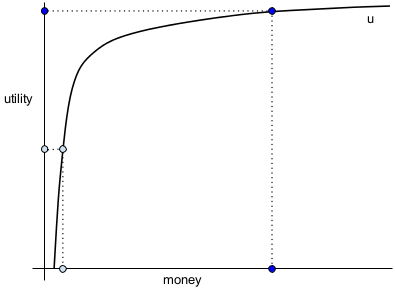Here is a gamble over two outcomes, higher value (dark blue) and lower value (light blue).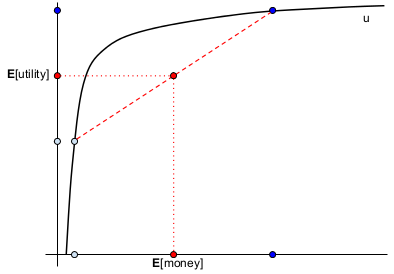Illustrates a 50% chance of each outcome, the corresponding expected monetary payoff, and the corresponding expected utility.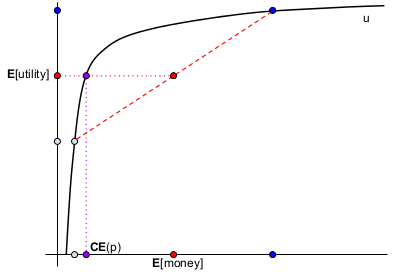The certainty equivalent ($CE$) of this gamble is the amount of money that, if obtained for certain, would have the same expected utility. This is much lower than the expected monetary payoff of the gamble, illustrating risk aversion.

It's beyond the scope of this article to discuss examples or literature in detail, but rest assured that risk-averse utility functions are very well studied both in general and in specific.

## Risk Aversion and Convexity

A further useful concept is the risk premium $RP^u(p) = \mathbb{E} X_p ~ - ~ CE^u(p)$. In other words, the agent would be indifferent to giving away the outcome of the lottery to an insurance company in return for $CE^u(p)$ for sure, whereupon the risk-neutral insurance company would make an expected profit of $RP^u(p)$.

Now, notice that risk aversion corresponds to $u^{-1}$ being convex (as $u$ is increasing and concave), so $RP^u(p)$ may look familiar -- it's just a "Jensen gap". (This means the difference in Jensen's inequality for a convex function - the reader should convince itself it understands this, looking up Jensen's Inequality if necessary). This ties in nicely with a definition of risk aversion that I personally prefer, in terms of convex functions of distributions.

Theorem. An agent is risk averse if and only if her certainty equivalent function $CE^u$ is convex.

Proof. Given the preceding discussion, we only need to show that $CE^u(p)$ is a convex function of $p$ if and only if $u^{-1}$ is a convex function of a single variable. The condition that $CE^u$ be convex is, for all $0 \leq \alpha \leq 1$ and all $p$, $q$, and $r = \alpha p + (1-\alpha) q$, we have $\alpha CE^u(p) + (1-\alpha)CE^u(q) \geq CE^u(r)$. This is $\alpha u^{-1}\left(\mathbb{E} X_p\right) + (1-\alpha) u^{-1}\left(\mathbb{E} X_q\right) \geq u^{-1}\left(\mathbb{E} X_r\right)$. The sneaky fact is that if we substitute $x = \mathbb{E} X_p$ and $y = \mathbb{E} X_q$, then we in fact have $z = \mathbb{E} X_r$ and this condition reads $\alpha u^{-1}(x) + (1-\alpha)u^{-1}(y) \geq u^{-1}(z)$, the condition that $u^{-1}$ be convex. $\square$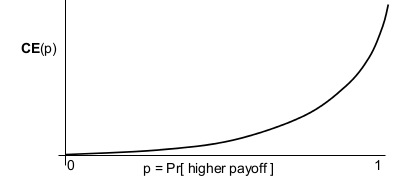Here we have a distribution with probability $p$ of a high payoff and $1-p$ of a low payoff (such as the dark and light blue points in the previous figure). On the $x$-axis is $p$, and the curve plots the certainty equivalent $CE(p)$, i.e. the agent is indifferent between the lottery $p$ and getting $CE(p)$ for sure.

Convexity intuitively corresponds to risk aversion because the payoff is higher on average at more extreme points, which correspond to more certainty in the lottery. The average utility of the outcomes is higher than the utility of facing the lottery.

## Risk Aversion and Value of Information

One way to think about risk aversion and lotteries is to imagine receiving a signal or piece of information about the outcome of the lottery. Then the previous theorem says the following

An agent is risk averse if and only if this signal makes them happier on average.

In other words, the better-informed version of themselves gets higher utility on average, just from being more certain. (For more and how to formalize this, please read my post on generalized entropy and value of information.)

A first natural answer could be to do with diminishing marginal utility for all those second-best things in life that money can buy. I can only eat about 500 Snickers bars per year, which translates to around $\$500$if buying in bulk. So my utility for money diminishes quickly past$\$500$ (as I only spend money on Snickers really, and a bit of diabetes insurance). A lottery ticket where I win $\$1000$with probability$0.5$is much less valuable to me than$\$500$: With the lottery ticket, half the time I get all the Snickers I want and half the time I get nothing, but with $\$500$for sure I always get just about all the Snickers I want. This is probably a reasonable explanation in many cases. But here, I want to suppose that an agent really is risk-neutral in the sense that her utility function is linear in money. However, we will model an addtional factor that makes her preferences over gambles risk-averse. ## Decisionmaking Suppose that the agent has a set of decisions available. Each of these decisions can result in a good or bad outcome depending on the outcome of the lottery. There is a simple explanation for why a risk-neutral agent becomes risk averse in such a setting. Under high uncertainty, the agent cannot make a very useful decision. But under low uncertainty, the agent can make a decision tailored to the likely outcome of the lottery, with good certainty that it will be the correct one. The agent has to pay a premium for facing risk. Example. Suppose I own the "half-chance$\$1000$, half-chance nothing" lottery ticket, with the outcome being revealed in one week. I would like to go to the Adele concert tomorrow, but tickets are currently $\$500$for a bad seat and$\$1000$ for a good seat. I am risk-neutral and value each seat at twice its price.

My options are to (a) not go to the concert; (b) take out a $\$500$loan and go with a bad seat; (c) take out a$\$1000$ loan and go with a good seat.

Unfortunately, the consequences of defaulting on my loan are so horrible that I choose option (a).

Now, if instead of the lottery ticket, I knew that in one week I would get $\$500$for certain, then I would have been able to take out the small loan and go to the concert, sitting in a bad seat. Thus, here although my utility for money is linear, my preferences demonstrate risk aversion: more certainty over the outcomes of my lottery allow me to make better decisions. Formally, suppose the agent's eventual monetary payoff depends both on the lottery outcome$\omega$and the decision she makes$d$, via the function$m(d,\omega)$. Let us suppose that the agent is technically risk-neutral in that her utility function$u(m) = \alpha m + \beta$for some constants$\alpha, \beta. Finally, suppose that the agent always takes the optimal decision for any given lottery, so she obtains utility $$G^u(p) := \max_d \mathbb{E}_p u(m(d, \omega)) .$$ and therefore her certainty equivalent becomes \begin{align*} CE^u(p) &:= u^{-1}\left( G^u(p) \right) \\ &= \frac{G^u(p) - \beta}{\alpha} , \end{align*} in other words, an affine function ofG^u$. Theorem. A decisionmaking agent with affine, "risk neutral" utility$u(m)$is risk averse in the sense that$CE^u$is convex. Proof.$G^u(p)$is a pointwise maximum of linear functions, hence is a convex function of$p$;$CE^u$is an affine function of this. In other words, imagine that we knew nothing about the decision problem the agent was facing, and we asked the agent to rank all lotteries from most-preferred to least-preferred. Then we would say that her preferences demonstrate risk aversion, i.e. if the lottery is on outcomes of money, her preferences would be explained by a concave function$u(m)$. Example. On a cold November day, Axl Rose and I are getting paid$\$100$ to carry a candle from location $A$ to $B$. We can either walk for free, or pay a vehicle to transport us for $\$30$. If we walk and it snows on us, we lose 20 utility. If we walk and it rains on us, we lose 80 utility. Our utility function is$u(m) = m$.  Action Weather Net utility Walk Clear 100 Walk Snow 80 Walk Rain 20 Ride Clear 70 Ride Snow 70 Ride Rain 70 Now if there is a one-third chance of each meteorological event, then we prefer to ride (expected utility$70$versus$66.66$). So our certainty equivalent for the all-one-third lottery is$\$70$. But now compare to an optimally informed version of ourselves: with probability one-third, it is clear and they walk; with one-third, it snows and they walk; with one-third, it rains and they ride.

These versions of us get expected utility $\frac{1}{3}100 + \frac{1}{3}80 + \frac{1}{3}70 = 83.33$. So we pay a "risk premium" of $\$13.33$. Illustration of example.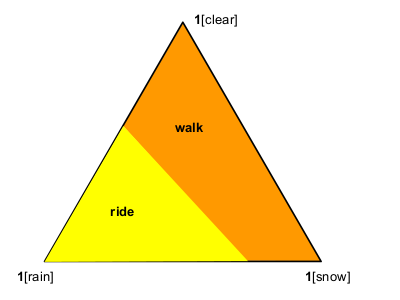Here we have a probability simplex on the three outcomes. Each point in the simplex is a lottery, i.e. a distribution on the outcomes. If the lottery we face lies in the orange space, the best decision for us is to walk; in the yellow, we should ride.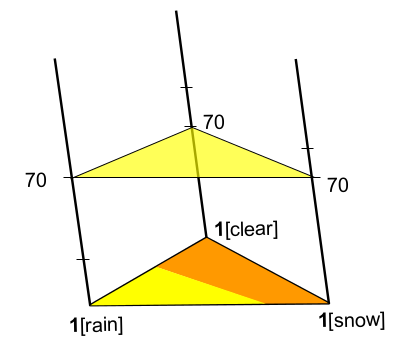Here is the expected utility on each point in the space if we ride: always 70.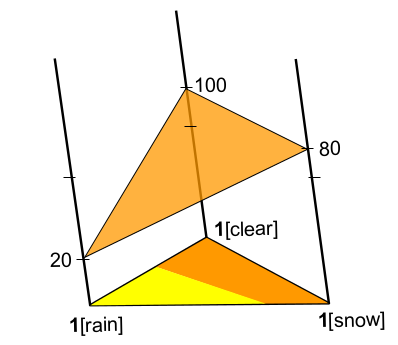Expected utility on each point if we walk; varies depending on the lottery.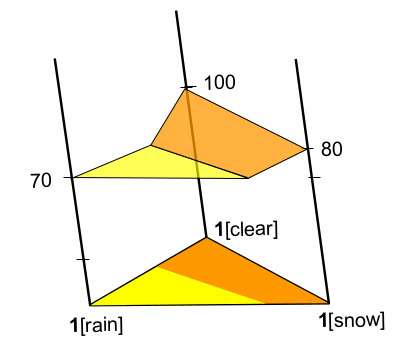Actual expected utility, also the certainty equivalent function in this example, for each lottery (assuming we act optimally given the lottery). This certainty equivalent is convex, hence Axl and I, despite quaslinear utility functions, demonstrate traits of risk aversion. ## Comments Post a comment: Sentient beings: Ignore the following two fields. Name: Limits to 1000 characters. HTML does not work but LaTeX does, e.g.$\$$x^y\$$ displays as $x^y$.
Please allow a few seconds after clicking "Submit" for it to go through.